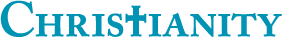## Voting Comparisons arranged by Day of the Week

0

(click on this box to dismiss)

Show ratio of up votes to down votes and average votes per post for both questions and answers arranged according to the day of the week.Q&A for committed Christians, experts in Christianity and those interested in learning more

``````-- Voting Comparisons arranged by Day of the Week

SELECT
DATENAME(WEEKDAY, p.CreationDate) AS Day,
CASE WHEN PostTypeId = 1 THEN 'Questions' ELSE 'Answers' END As [Post Type],
Count(*) AS [Post Count],
SUM(CASE WHEN VoteTypeId = 2 THEN 1 ELSE 0 END) AS [Up Votes],
SUM(CASE WHEN VoteTypeId = 3 THEN 1 ELSE 0 END) AS [Down Votes],
ROUND((CAST((SUM(CASE WHEN VoteTypeId = 2 THEN 1 ELSE 0 END) + SUM(CASE WHEN VoteTypeId = 3 THEN 1 ELSE 0 END)) AS float)) / COUNT(p.Id), 4) AS [Votes per Post],
ROUND((CAST(SUM(CASE WHEN VoteTypeId = 2 THEN 1 ELSE 0 END) AS float) / CAST(SUM(CASE WHEN VoteTypeId = 3 THEN 1 ELSE 0 END) AS float)), 4) AS [Up vs Down Ratio]

FROM
Votes v JOIN Posts p ON v.PostId=p.Id
GROUP BY
PostTypeId, DATEPART(WEEKDAY, p.CreationDate), DATENAME(WEEKDAY, p.CreationDate)
ORDER BY
DATEPART(WEEKDAY, p.CreationDate), PostTypeId``````

### Enter Parameters

Options:
-Hold tight while we fetch your results
:records returned in :time ms:cached